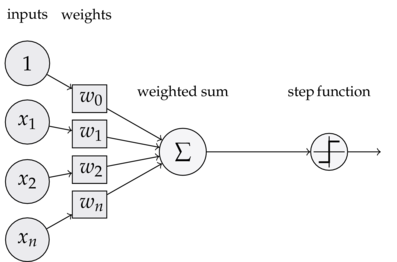Perceptron Learning rule

February 07, 2019

The Perceptron Learning Rule was really the first approaches at modeling the neuron for learning purposes. It was based on the MCP neuron model.

This article tries to explain the underlying concept in a more theoritical and mathematical way.

The whole idea behind MCP neuron model and the perceptron model is to minimally mimic how a single neuron in the brain behaves. it either fires or doesn’t fire.

The perceptron rule is thus, fairly simple, and can be summarized in the following steps:-

1) Initialize the weights to 0 or small random numbers.

2) For each training sample x^(i):
* Compute the output value y^
* update the weights based on the learning rule

Terminology and components of the Perceptron

The Perceptron learning rule:-

1. Input Vector / Input matrix / Input values:- the data values as vectors/matrix/rows

2. Weight Vector:- a column vector containing weights for each dimension of input value.

3. Net input:- The linear combination of the input values (x) and the weight vector (w)

the net input $z = w_{1}x_{1} + w_{2}x_{2} + ... + w_{m}x_{m}$

$w = \begin{bmatrix}w_{1} \\ .\\.\\.\\w_{m} \end{bmatrix} , x = \begin{bmatrix}x_{1}\\.\\.\\.\\x_{m}\end{bmatrix} \\ \\ z\ = \ w_{1}x_{1} + w_{1}x_{1} + ... + w_{m}x_{m}$

Now, in context of binary classification task, if the net input of a particular sample $x_{i}$ is greater than a defined threshold θ, we predict class 1, else class -1.

In case of perceptron, the decision function is a variant of the step function.

$\phi$(z) = 1 if z >= θ, -1 otherwise.

for simplicity, we can bring the threshold θ to the left size of the equation and define a weight-zero as w{0}_ = - θ and x0 = 1, so that we can write in a more compact form:

$z = w_{0}x_{0} + w_{1}x_{1} + .... + w_{m}x_{m} = x^T w$

and

$\phi$(z) = 1 if z>= 0, -1 otherwise

In ML, this negative threshold, or weight, $w_{0}$ = -θ, is usually called bias unit

Diagram:After each sample, all the weights in the weights vector are updated, according to the rule:-

$w_{j} := w_{j} + \Delta w_{j}$ where,

$\\ \Delta w_{j} = \eta (y^{(i)} - \hat y^{(i)})x^{(i)}_{j}$

where,

$y^{(i)} = actual\ output$ $\\ \hat y^{(i)} = predicted\ output$ $\\ \eta = learning\ rate$

Catch

For all the simplicity the perceptron rule offers, theres a catch to it when applying it for binary classification: -

The convergence[] of perceptron is only achived if the two classes are linearly seprable, i.e. can be separated by a linear decision boundary.

• we can:-

1. Set the max number of passes of dataset(epochs)
2. Set a threshold for max number of misclassifications

else, the perceptron will never stop updating.